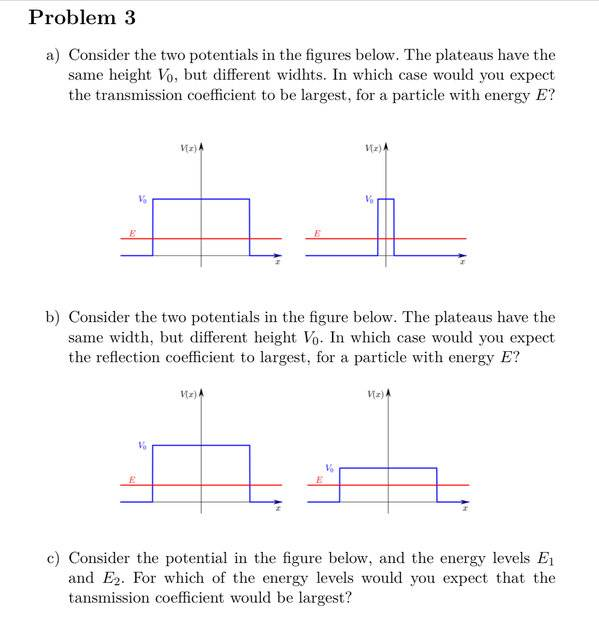# Quantum mechanics - finite square well

• Graham87

#### Graham87

Homework Statement
In which case would you expect the reflection coefficient to largest, for a particle with energy E?

For which of the energy levels would you expect that the tansmission coefficient would be largest?
Relevant Equations
##R=\frac{|A|^2}{|B|^2}##

##T=1-R##

##k=\sqrt{\frac{2m(V_0-E)}{h}}##

##\psi(x)=Ae^{kx}+Be^{-kx}##In a) I get that T should be largest where V_0 is least wide, because when V_0 is infinitely wide the particle would be fully reflected.
But I don't get how height in b) and energy levels height in c) correlates to T and R.

Is it because of their k? I get the opposite answer from the correct one when I plug k in the R formula.

##R=\frac{|A|^2}{|B|^2}##

##T=1-R##

##k=\sqrt{\frac{2m(V_0-E)}{h}}##

##\psi(x)=Ae^{kx}+Be^{-kx}##

Thanks!

Last edited:

•